Want to share your content on R-bloggers? click here if you have a blog, or here if you don't.

I recently tried to answer a simple question, asked by @adelaigue. Actually, I thought that the answer would be obvious… but it is a little bit more compexe than what I thought. In a recent pool about elections in Brazil, it was mentionned in a French newspapper that “Mme Rousseff, 62 ans, de 46,8% des intentions de vote et José Serra, 68 ans, de 42,7%” (i.e. proportions obtained from the survey). It is also mentioned that “la marge d’erreur du sondage est de 2,2% ” i.e. the margin of error is 2.2%, which means (for the journalist) that there is a “grande probabilité que les 2 candidats soient à égalité” (there is a “large probability” to have equal proportions).
Usually, in sampling theory, we look at the margin of error of a single proportion. The idea is that the variance of widehat{p}, obtained from a sample of size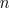isthus, the standard error isThe standard 95% confidence interval, derived from a Gaussian approximation of the binomial distribution isThe largest value is obtained when p is 1/2, and then we have a worst case confidence interval (an upper bound) which isSo with a margin of errormeans that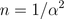. Hence, with a 5% margin of error, it means that n=400. While 2.2% means that n=2000:
> 1/.022^2
 2066.116

Classically, we compare proportions between two samples: surveys at two different dates, surveys in different regions, surveys paid by two different newpapers, etc. But here, we wish to compare proportions within the same sample. This has been consider in an “old” paper published in 1993 in the American Statistician,It contains nice figures to illustrate the difference between the standard approach,and the one we would like to study here.This point is mentioned in the book by Kish, survey sampling (thanks Benoit for the reference),Let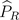anddenote empirical frequencies we have obtained from the sample, based onobservations. Then since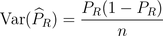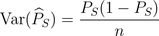andwe have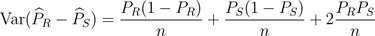Thus, a natural margin of error on the difference between the two proportion is herewhich is here 4 points
> n=2000
> p1=46.8/100
> p2=42.7/100
> 1.96*sqrt((p1+p2)-(p1-p2)^2)/sqrt(n)
 0.04142327
Which is exactly the difference we have here ! Hence, the probability of reaching such a value is quite small (2%)
> s=sqrt(p1*(1-p1)/n+p2*(1-p2)/n+2*p1*p2/n)
> (p1-p2)/s
 1.939972
> 1-pnorm(p1-p2,mean=0,sd=sqrt((p1+p2)-(p1-p2)^2)/sqrt(n))
 0.02619152Actually, we can compare the three margin of errors we have so far,
• the upper bound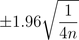• the “average one”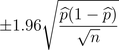where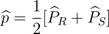• the more accurate one we just obtained,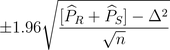where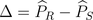.
> p=seq(0,.5,by=.01)
> ic1=rep(1.96/sqrt(4*n),length(p))
> ic2=1.96*sqrt(p*(1-p))/sqrt(n)
> delta=.01
> ic31=1.96*sqrt(2*p-delta^2)/sqrt(n)
> delta=.2
> ic32=1.96*sqrt(2*p-delta^2)/sqrt(n)
> plot(p,ic32,type=”l”,col=”blue”)
> lines(p,ic31,col=”red”)
> lines(p,ic2)
> lines(p,ic1,lty=2)
So on the graph below, the dotted line is the standard upper bound, the plain line in black being a more accurate one when the probability is(the x-axis). The red line is the true margin of error with a large difference between candidates (20 points) and the blue line with a small difference (1 point).Remark: an alternative is to consider a chi-square test, comparering two multinomial distributions, with probabilitiesandwhereis the average proportion, i.e. 44.75%. Then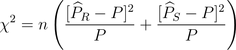i.e.=3.71
> p=(p1+p2)/2
> (x2=n*((p1-p)^2/p+(p2-p)^2/p))
 3.756425
> 1-pchisq(x2,df=1)
 0.05260495
Under the null hypothesis,should have a chi-square distribution, with one degree of freedom (since the average is fixed here). Here the probability to reach that level is around 5% (which can be compared with the 2% we add before).So finally, I would think that here, stating that there is a “large probability” is not correct….